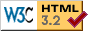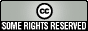# JDEdwards - P98308Q - Build DreamWriter OPNQRYF statement

Each DreamWriter allows us to specify sequence and selection. This information is used to create a OPNQRYF statement (on the DreamWriter Versions screen, an option 7 will show us the created OPNQRYF). If the Based On file contains MCU, then Business Unit security will be incorporated into the selection criteria as well. If the QRYSLT clause of the OPNQRYF statement grows too large, then P98308Q will terminate abnormally with an array index error.

If you make extensive use of Business Unit Security, this could happen with frustrating regularity. But there are a couple of things we can do to shrink the size of the QRYSLT clause.

One of our opportunities for improvement is that all of the Business Unit Security selections are expressed in %RANGE subclauses.

```
OPNQRYF FILE((F0311A)) OPTION(*INP) FORMAT(*FILE) OPTIMIZE(*ALLIO) OPNID(F0311)
TYPE(*PERM) KEYFLD((RPCO) (RPAN8) (RPALPH)) QRYSLT('((RPMCU  = " ")  |
(RPMCU  = %RANGE("           1" "           1")) |
(RPMCU  = %RANGE("        ELEC" "        ELEC")) |
(RPMCU  = %RANGE("        FILT" "        FILT")) |
(RPMCU  = %RANGE("        MECH" "        MECH")) |
(RPMCU  = %RANGE("        S600" "        S724")) |
(RPMCU  = %RANGE("        WARE" "        WARE")) |
(RPMCU  = %RANGE("        WELD" "        WELD")) |
(RPMCU  = %RANGE("        0121" "        0121")) |
(RPMCU  = %RANGE("        0124" "        0124")) |
(RPMCU  = %RANGE("        0600" "        0610")) |
(RPMCU  = %RANGE("        0700" "        0724")) |
(RPMCU  = %RANGE("        0950" "        0950")) |
(RPMCU  = %RANGE("        1406" "        1408")) |
(RPMCU  = %RANGE("        1410" "        1410")) |
(RPMCU  = %RANGE("        1420" "        1420")) |
(RPMCU  = %RANGE("        8500" "        8539")) |
(RPMCU  = %RANGE("        8541" "        8541")) |
(RPMCU  = %RANGE("        8543" "        8599")) |
(RPMCU  = %RANGE("        8701" "        8705")) |
(RPMCU  = %RANGE("        8800" "        8816")) |
(RPMCU  = %RANGE("        8852" "        8901")) |
(RPMCU  = %RANGE("     AFE0000" "     AFE0000")) | ... etc.
```

Whew! We are wasting a tremendous amount of space specifying ranges around a single value. Suppose we could replace them with straight equal arguments?

```OPNQRYF FILE((F0311A   )) OPTION(*INP) FORMAT(*FILE) OPTIMIZE(*ALLIO  ) OPNID(F0311)
TYPE(*PERM) KEYFLD((RPCO  ) (RPAN8 ) (RPALPH)) QRYSLT('((RPMCU  = " ")  |
(RPMCU  = "           1") |
(RPMCU  = "        ELEC") |
(RPMCU  = "        FILT") |
(RPMCU  = "        MECH") |
(RPMCU  = %RANGE("        S600" "        S724")) |
(RPMCU  = "        WARE") |
(RPMCU  = "        WELD") |
(RPMCU  = "        0121") |
(RPMCU  = "        0124") |
(RPMCU  = %RANGE("        0600" "        0610")) |
(RPMCU  = %RANGE("        0700" "        0724")) |
(RPMCU  = "        0950") |
(RPMCU  = %RANGE("        1406" "        1408")) |
(RPMCU  = "        1410") |
(RPMCU  = "        1420") |
(RPMCU  = %RANGE("        8500" "        8539")) |
(RPMCU  = "        8541") |
(RPMCU  = %RANGE("        8543" "        8599")) |
(RPMCU  = %RANGE("        8701" "        8705")) |
(RPMCU  = %RANGE("        8800" "        8816")) |
(RPMCU  = %RANGE("        8852" "        8901")) |
(RPMCU  = "     AFE0000") ... etc.
```

Just replacing the %RANGE subclauses with straight equals has saved us a bunch of space; possibly enough to keep from killing the P98308Q. So, how do we replace these %RANGES? Easily. In fact, the original developers practically show us how

```      C*
C*   If *BLANK to *BLANK, output shorter phrase.
C*
CSR         MSMCUF    IFEQ *BLANKS
CSR         MSMCUT    ANDEQ*BLANKS
CSR                   MOVEA'" ")'    OQF,#Z
CSR                   ELSE
addedC*   If MCUF = MCUT, output shorter phrase.
C*
C*   Output a %RANGE phrase.
C*
CSR                   MOVEA'%RANGE("'OQF,#Z
C*
DBN1 CSR                   MOVEAMSMCUF    OQF,#Z
C*
CSR                   MOVEA'" "'     OQF,#Z
C*
DBN1 CSR                   MOVEAMSMCUT    OQF,#Z
C*                                                                      CSR                   MOVEA'"))'     OQF,#Z